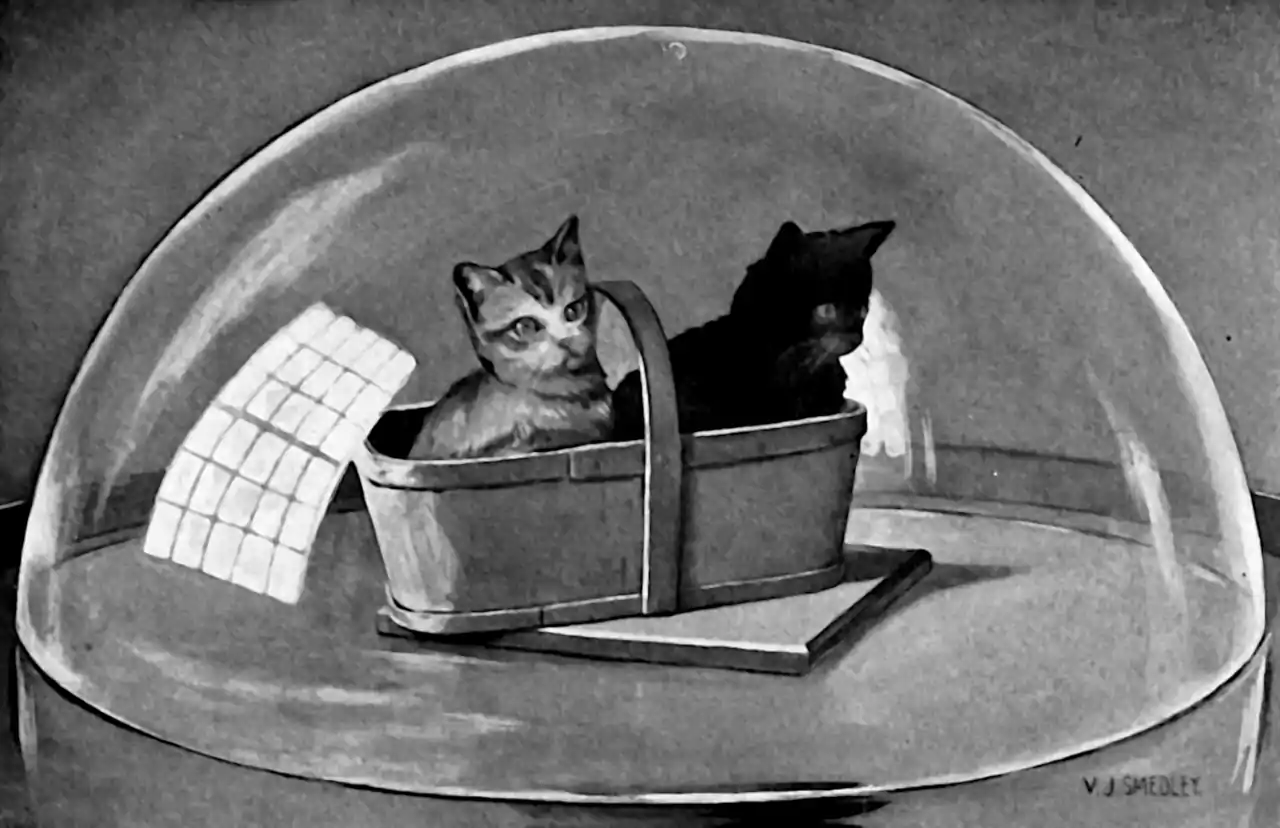# High dimensional statistics

Placeholder to think about the many weird problems arising in very high dimensional statistical inference. There are many approaches to this problem: throwing out dimensions/predictors as in model selection, considering low dimensional projections, viewing objects with matrix structure for concentration or factorisation, or tensor structure even.

## Soap bubbles

High dimensional distributions are extremely odd, and concentrate in weird ways. For example, for some natural definitions of typical, typical items are not average items in high See Sander Dielemann’s musings on typicality for an introduction to this plus some motivating examples.

For another example, consider this summary result of Vershynin (2015):

Let $$K$$ be an isotropic convex body (e.g. an $$L_2$$ ball) in $$\mathbb{R}^{n},$$ and let $$X$$ be a random vector uniformly distributed in $$K$$, with $$\mathbb{E}X=0$$ and $$\mathbb{E}XX^{\top}=I_n.$$ Then the following is true for some positive constants $$C,c$$:

1. (Concentration of volume) For every $$t \geq 1$$, one has $\mathbb{P}\left\{\|X\|_{2}>t \sqrt{n}\right\} \leq \exp (-c t \sqrt{n})$
2. (Thin shell) For every $$\varepsilon \in(0,1),$$ one has $\mathbb{P}\left\{\left|\|X\|_{2}-\sqrt{n}\right|>\varepsilon \sqrt{n}\right\} \leq C \exp \left(-c \varepsilon^{3} n^{1 / 2}\right)$

That is, even with the mass uniformly distributed over space, as the dimension grows, it all ends up in a thin shell, because volume grows exponentially in dimension. This is popularly known as a soap bubble phenomenon. This is one of the phenomena that leads to interesting behaviour in low dimensional projection. The more formal name is the Gaussian Annulus Theorem. Turning it around, for a d-dimensional spherical Gaussian with unit variance in each direction, for any $$\beta \leq \sqrt{d}$$, all but at most $$3 e^{-c \beta^{2}}$$ of the probability mass lies within the annulus $$\sqrt{d}-\beta \leq|\mathbf{x}| \leq \sqrt{d}+\beta,$$ where $$c$$ is a fixed positive constant.## Convex hulls

Balestriero, Pesenti, and LeCun (2021) cite Bárány and Füredi (1988):

Given a $$d$$-dimensional dataset $$\boldsymbol{X} \triangleq\left\{\boldsymbol{x}_{1}, \ldots, \boldsymbol{x}_{N}\right\}$$ with i.i.d. samples $$\boldsymbol{x}_{n} \sim \mathcal{N}\left(0, I_{d}\right), \forall n$$, the probability that a new sample $$\boldsymbol{x} \sim \mathcal{N}\left(0, I_{d}\right)$$ is in interpolation regime (recall Def. 1 ) has the following limiting behavior $\lim _{d \rightarrow \infty} p(\underbrace{\boldsymbol{x} \in \operatorname{Hull}(\boldsymbol{X})}_{\text {interpolation }})= \begin{cases}1 & \Longleftrightarrow N>d^{-1} 2^{d / 2} \\ 0 & \Longleftrightarrow N<d^{-1} 2^{d / 2}\end{cases}$

They observe that this implies high dimensional statistics rarely interpolates between data points, which is not surprising, but only in retrospect. Despite some expertise in high-dimensional problems I had never noticed this fact myself. Interestingly they collect evidence that suggests that low-d projections and latent spaces are also rarely interpolating.

## Empirical processes in high dimensions

Combining empirical process theory with high dimensional statistics gets us to some interesting models. See, e.g. van de Geer (2014b).

## Markov Chain Monte Carlo in high dimensions

TBD

### No comments yet. Why not leave one?

GitHub-flavored Markdown & a sane subset of HTML is supported.